# Slopes Between Points on a Line

Alignments to Content Standards: 8.EE.B.6

The slope between two points is calculated by finding the change in $y$-values and dividing by the change in $x$-values. For example, the slope between the points (7, -15) and (-8, 22) can be computed as follows:

• The difference in the $y$-values is $-15-22 = -37$.
• The difference in the $x$-values is $7-(-8) = 15$.
• Dividing these two differences, we find that the slope is $-\frac{37}{15}$.
• Eva, Carl, and Maria are computing the slope between pairs of points on the line shown below.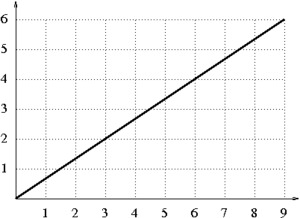Eva finds the slope between the points (0,0) and (3,2). Carl finds the slope between the points (3,2) and (6,4). Maria finds the slope between the points (3,2) and (9,6). They have each drawn a triangle to help with their calculations (shown below).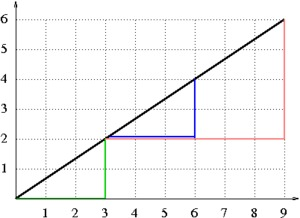1. Which student has drawn which triangle? Finish the slope calculation for each student. How can the differences in the $x$- and $y$-values be interpreted geometrically in the pictures they have drawn?
2. Consider any two points $(x_1, y_1)$ and $(x_2, y_2)$ on the line shown above. Draw a triangle like the triangles drawn by Eva, Carl, and Maria. What is the slope between these two points? Why should this slope be the same as the slopes calculated by the three students?

## IM Commentary

The "change in $y$ divided by the change in $x$" can be computed for any two points in the plane. Many people who understand and use slope take for granted the fact that the slopes between any two points on a particular line will always be equal--most of us just learned it as a fact. The purpose of this task is to help students understand why the calculated slope will be the same for any two points on a given line. This is the first step in understanding and explaining why it will work for any line (not just the line shown).

In 8th grade, students describe the effect that dilations have on a figure (see 8.G.A.3). They also learn that if one triangle can be obtained from another by a series of translations and dilations, then they are similar (see 8.G.A.4). Putting these two pieces of information together, they can argue that for any two points on a line, the "slope triangles" (like the ones shown in the figure in the task statement) have to be similar, and as a result the lengths of the sides of the triangles will be proportional. This is why the slope between any two points on a particular line will always be equal, and why we talk about "the" slope of a line.

This instructional task is intended to be used in a class discussion. Students can work on parts (a) and (b) independently or in small groups. Then the class could discuss their answers and discuss part (c). Students should be given a chance to try to construct the argument for part (c) on their own, although some may struggle with this. It is important that there is a whole-class discussion of this part so that everyone understands the argument in the end.

## Solution

1. Eva is using the green triangle, since two of the vertices of the triangle are at $(0,0)$ and $(3,2)$. She will next find the length of each leg in the right triangle. The horizontal leg length is the difference between the $x$-coordinates, which is 3. The vertical leg length is the difference between the $y$-coordinates, which is 2. So the line rises by 2 units for every horizontal increase of 3 units. Therefore the slope is $\frac{2}{3}$.Carl is using the blue triangle, since two of the vertices of the triangle are at $(3,2)$ and $(6,4)$. He will next find the length of each leg in the right triangle. The horizontal leg length is the difference between the $x$-coordinates, which is 3. The vertical leg length is the difference between the $y$-coordinates, which is 2. So the line rises by 2 units for every horizontal increase of 3 units. Therefore the slope is $\frac{2}{3}$.

Maria is using the red triangle, since two of the vertices of the triangle are at $(3,2)$ and $(9,6)$. She will next find the length of each leg in the right triangle. The horizontal leg length is the difference between the $x$-coordinates, which is 6. The vertical leg length is the difference between the $y$-coordinates, which is 4. So the line rises by 4 units for every horizontal increase of 6 units. Therefore, the slope is $\frac{4}{6}$.

2. To compute the slope between two points, we are computing the quotient of the lengths of the legs in a right triangle. We can see that the blue and the green triangles are congruent since we can translate the green triangle along the line until it lines up with the blue triangle. Therefore, the quotient of the lengths of the legs must be the same.The red triangle is not congruent to the blue triangle but it is similar to it. We can dilate the blue triangle by a factor of 2 to line it up with the red triangle. The sides in similar triangles also have the same proportion. Therefore, the quotient of the lengths of the legs of the two triangles must be the same.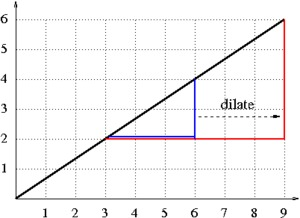3. Parts (a) and (b) suggest the following picture: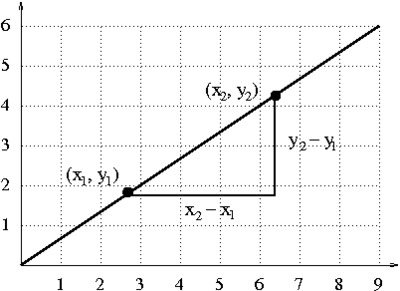The lengths of the vertical leg of this triangle is $y_2-y_1,$ and the length of the horizontal leg is $x_2-x_1$.

The slope between these two points is the quotient of these two lengths: $\frac{y_2-y_1}{x_2-x_1}$.

This slope should be the same as the slope obtained by Eva because her green triangle is similar to the triangle we drew above. To see this,

• First, translate Eva's triangle $x_1$ units to the right and $y_1$ units up. Now the two triangles share a vertex.
•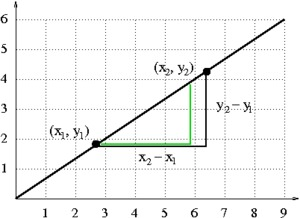• Next, dilate Eva's triangle by a factor of $\frac13$ (so the horizontal leg has a length of 1) and then by a factor of $x_2-x_1$ (so the horizontal leg has a length of $x_2-x_1$). Use the common vertex as the center of the dilation.
•Because angles are preserved by translations and dilations, this shows that Eva's triangle and our triangle are similar and that the legs are proportional. So $$\frac{y_2-y_1}{x_2-x_1}=\frac23$$ and the slope is the same no matter which two points on this line we choose to compute with.